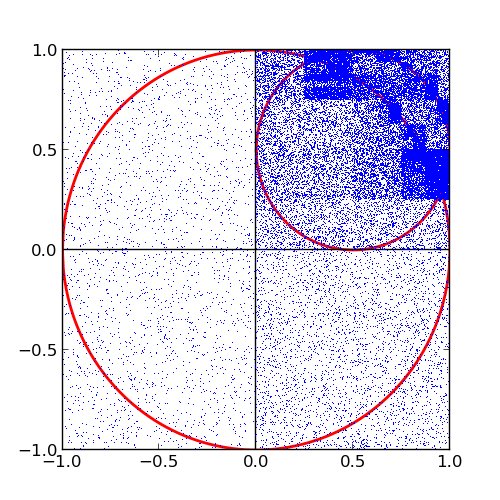A friend of mine sent me the picture below recently. This is my solution.I thought about it for a second and then remembered that three years ago I wrote a script to deal with this kind of problem. As part of a course on numerical methods I wrote a Monte Carlo integrator to integrate two-dimensional functions.

In this case we are dealing with the two circles parametrised by $x^2+y^2 = 1$ $\left(x-\frac{1}{2}\right)^2+\left(y-\frac{1}{2}\right)^2 = \frac{1}{4}$

We find the intersecting points for these two functions and call the associated x-coordinates $x_1$ and $x_2$. Our area of interest is then parametrised by

$f(x,y) = 1, \text{ if } \begin{cases}x_1 < x < x_2\\\sqrt{1-x^2} < y < \sqrt{x^2-x+\frac{1}{4}}+\frac{1}{2}\end{cases}$

Now it is only a matter of feeding the intersections together with this parametrised function to the integrator. What the integrator does is basically this

$A = \int_{-1}^1\int_{-1}^1f\,dxdy.$

The result of the stratified Monte Carlo integration (with 50000 sample points) can be seen in the figure below. The area of the region of interest is $0.14628 \pm 0.00008$ with $10^6$ sampled points. Since I am not a mathematician this is an acceptable result.I put the source to the solution on GitHub.

Edit: In August 2014 I was contacted by Bruce M. Boghosian, PhD, Tufts University, who found an error in my integral. I have updated the results on this page and also the source on GitHub.

Edit: In February 2015 I was contacted by Laird Shaw, who has found a definite integral to solve the problem. You can evaluate it here.